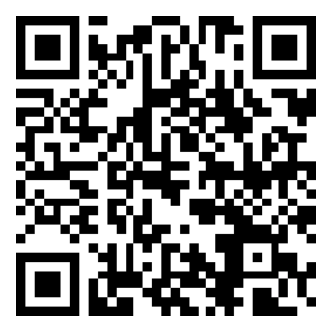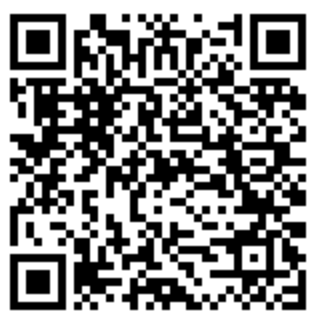# Zoho Deluge - Generate 5 Letter Booking Retrieval Code

What?
This is a quick article on how to generate a 5 letter code from several functions: one which returns 5 randomly selected characters and another two which convert a number to 5 letters.

Why?
Zoho doesn't have a function to generate random numbers or strings. There are workarounds however.

How?
The first note is that there is something that can emulate a random number... well it's not really random, it involves getting the milliseconds from a time object.
```     v_Uid = zoho.currenttime.toLong();
// returns something like 1557400230165
```

Method #1: 5 Randomly Selected Letters

Using a snippet from the community forums on how someone shuffled a pack of cards, I came up with a function that will take a list of letters, shuffle the list, and return the first 5 characters.
```string generateRetrievalCode()
{
// init
v_Output = "";
l_Output = List:String();
l_Alphabet = {"A","B","C","D","E","F","G","H","I","J","K","L","M","N","O","P","Q","R","S","T","U","V","W","X","Y","Z"};
v_StrLength = 5;

// everyday I'm shufflin
l_OutputShuffled = List();
v_OutputListSize = l_Alphabet.size();
for each v_Element in l_Alphabet
{
v_Random = (zoho.currenttime.toLong()  %  v_OutputListSize);
l_Alphabet.remove(v_Random);
v_OutputListSize = v_OutputListSize - 1;
}
l_Output = l_OutputShuffled.subList(0,v_StrLength);
v_Output= l_Output.toString().replaceAll(",","");
return v_Output;
}
```
This is my preferred method and it will return a string of 5 random uppercase letters.

Method #2: Convert time to letters
I've previously used substring to extract the milliseconds into 5 numbers and get the remainder from dividing it by 26 (modulus)... This is NOT to be used for Booking Retrieval Codes as it is easy to determine what code if you have the date/time of the booking as well as the customer's surname (method #1 would not be guessed by date/time):
```string generateRetrievalCode()
{
// init
v_Output = "";
l_Output = List:String();
l_Alphabet = {"A","B","C","D","E","F","G","H","I","J","K","L","M","N","O","P","Q","R","S","T","U","V","W","X","Y","Z"};
v_StrLength = 5;

// convert milliseconds into 5 parts
v_Uid = zoho.currenttime.toLong();
v_UidStr = v_Uid.toString().trim();

// loop through parts
for each v_Element in l_Parts
{
v_Remainder = v_Element.toLong() % 26;
}

// output
v_Output= l_Output.toString().replaceAll(",","");
return v_Output;
}
```

Method #3: randomNumber() function
Using the randomNumber() function we can hope this works reliably:
```string generateRetrievalCode()
{
// init
v_Output = "";
l_Output = List;
l_Alphabet = {"A","B","C","D","E","F","G","H","I","J","K","L","M","N","O","P","Q","R","S","T","U","V","W","X","Y","Z"};
l_StrLength = {0,1,2,3,4};

// assign a letter to a list entry
for each index  v_Index in l_StrLength
{
}

// output
v_Output= l_Output.toString().replaceAll(",","");
return v_Output;
}
```

As this was for a client who arranged outdoor activities for kids, I need to add a filter which blocks out swear words and the such. Experimental - DO NOT USE:
```string generateRetrievalCode()
{
// ... refer to above functions
}
string generateKidSafeRetrievalCode()
{
// init
v_Output = "";
l_TimesTried = {1,2,3,4,5};

// loop through times you will chance it
for each v_TimeTried in l_TimesTried
{
// init

// get attempt
v_Output = thisapp.generateRetrievalCode();

// see if can use contains but check for word
{
{
}
}

{
break;
}
}

// output
return v_Output;
}
```

Source(s):

### Accreditation### Donate & Support

If you like my content, and would like to support this sharing site, feel free to donate using a method below:

Paypal:Bitcoin:3QnhmaBX7LQSRsC9hh6Je9rGQKEGNQNfPb